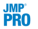Publication date: 11/10/2021

#Singular Value Decomposition

Singular value decomposition (SVD) complements association analysis by providing another method to identify items that have an affinity for each other. Singular value decomposition of the transaction item matrix reduces the matrix to a manageable number of dimensions, thereby enabling you to group similar transactions and similar items. The SVD analysis is equivalent to performing principal components analysis (PCA) on a correlation matrix.

The transaction item matrix is a matrix for which each row corresponds to a transaction and each column corresponds to an item. The entries of the matrix are zeros and ones. If an item occurs in a transaction, the corresponding row and column entry is one. Otherwise, the row and column entry is zero. Because the transaction item matrix usually contains more values of zero than one, it is called a sparse matrix.

The partial singular value decomposition approximates the column-standardized transaction item matrix using three matrices: U, S, and V. The relationship between these matrices is defined as follows:

Transaction Item Matrix U * S * V

Define nTran as the number of transactions (rows) in the transaction item matrix, nItem as the number of items (columns) in the transaction item matrix, and nVec as the specified number of singular vectors. Note that nVec must be less than or equal to min(nTran, nItem). It follows that U is an nTran by nVec matrix that contains the left singular vectors of the transaction item matrix. S is a diagonal matrix of dimension nVec. The diagonal entries in S are the singular values in the transaction item matrix. V is an nVec by nItem matrix. The rows in V (or columns in V) are the right singular vectors.

The right singular vectors capture connections among different items with similar functions or topic areas. If three items tend to appear in the same transactions, the SVD is likely to produce a singular vector in V with large values for those three items. The U singular vectors represent the transactions projected into this new item space.

The SVD also captures indirect connections. If two items never appear together in the same transaction, but they generally appear in transactions with another third item, the SVD is able to capture some of that connection. If two transactions have no items in common but contain items that are connected in the dimension-reduced space, they map to similar vectors in the SVD plots.

The SVD transforms transaction data into a fixed-dimensional vector space, making it amenable to clustering, classification, and regression techniques. The Save options enable you to export this vector space to be analyzed in other JMP platforms.

The transaction item matrix is centered, scaled, and divided by nTran minus 1 before the singular value decomposition is carried out. This analysis is equivalent to a PCA of the correlation matrix of the transaction item matrix. The SVD implementation takes advantage of the sparsity of the transaction item matrix.